# 60 and Pay No Attention to That Man Behind the Curtain

60 is a composite number. 60 = 1 x 60, 2 x 30, 3 x 20, 4 x 15, 5 x 12, or 6 x 10. Factors of 60: 1, 2, 3, 4, 5, 6, 10, 12, 15, 20, 30, 60. Prime factorization: 60 = 2 x 2 x 3 x 5, which can also be written 60 = 2² x 3 x 5.Sometimes 60 is a clue in the FIND THE FACTORS puzzles. Even though it has many other factors, we only use 6 x 10 for the FIND THE FACTORS 1-10 puzzles and 6 x 10 or 5 x 12 for the FIND THE FACTORS 1-12 puzzles.

When solving a level 6 puzzle, trying to place the numbers from 1 to 10 in the top row as well as the first column can be frustrating. Every single clue appears to have more than one possible answer. How can someone guess right with every single number placement? Is seeing the answer the only way to solve the puzzle?

Here is this week’s level 6 puzzle. Usually to solve a puzzle, you start with a row or column with 2 clues.  There are 6 rows or columns with more than one clue, and every one of them is no help!You can also find this and a few other puzzles in an excel file here. If you have a spreadsheet program on your computer, you can access it. If you enable editing in excel, you can type your answers directly onto the puzzle, and you can also easily print the puzzles.

“Pay no attention to the that man behind the curtain!” is a famous line from The Wizard of Oz.  Everyone in Oz thought the Wizard was powerful enough to solve any problem. When Dorthy and her friends looked behind the curtain they discovered that he was really an ordinary man who was able to find an advantage in what other people didn’t know. It turns out that other people actually had the abilities they thought only the Wizard had. Today we will take a peek behind the curtain of a level 6 puzzle and discover that it really isn’t as intimidating as we might imagine, and that you have the ability to solve such a puzzle, too. This is what last week’s level 6 puzzle looks like from behind the curtain:Many people try to start with the row that contains the clue 4 x 8 = 32. (When I solve this puzzle, that row is actually the one I FINISH with!) Instead look at the row with no clues. If you study all the clues in the puzzle, you will notice that 6 is listed as a factor only once. If there were a clue in the row without any clues, that clue would be a multiple of 6. That hint will keep you going for several clues. It also eliminates 8 as a possible factor of 72 and ensures that 1 x 8, not 2 x 4, must used for the clue 8. Which means that 2 x 5, not 1 x 10, must be used for the clue 10 because both ones will have been used. Which means that 2 x 10, not 4 x 5, must be used for the clue 20 because the number 10 has to be used as a factor twice. Here is the completed puzzle:# 59 and Valentine Gif

• 59 is a prime number.
• Prime factorization: 59 is prime.
• The exponent of prime number 59 is 1. Adding 1 to that exponent we get (1 + 1) = 2. Therefore 59 has exactly 2 factors.
• Factors of 59: 1, 59
• Factor pairs: 59 = 1 x 59
• 59 has no square factors that allow its square root to be simplified. √59 ≈ 7.6811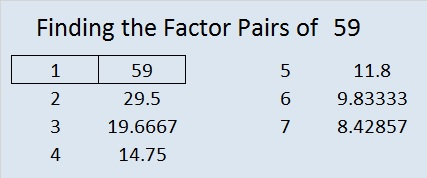How do we know that 59 is a prime number? If 59 were not a prime number, then it would be divisible by at least one prime number less than or equal to √59 ≈ 7.7. Since 59 cannot be divided evenly by 2, 3, 5, or 7, we know that 59 is a prime number.

59 is never a clue in the FIND THE FACTORS puzzles.Valentine’s Day is almost here. Here is a Valentine you can share with your friends who enjoy solving puzzles.

To solve the puzzle, the numbers 1 through 10 need to be written on the top row and also on the first column so that those numbers and the clues inside the puzzle make a multiplication table. Since it’s a level 5 puzzle, it may be a little challenging.

Your friend will not have to wait a whole week for the solution because there is a gif that will solve it quickly and logically. The gif could be the valentine, too.

This week’s puzzles are available in an excel file here. If you have a spreadsheet program on your computer, you can access it. If you enable editing in excel, you can type your answers directly onto the puzzle, and you can also easily print the puzzles.

Here are the factors to last week’s puzzle.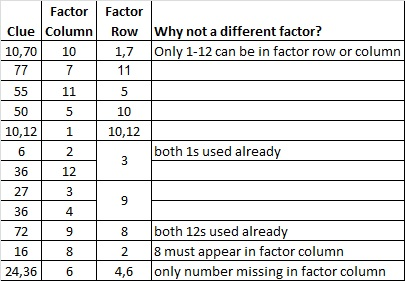<a data-pin-do=”embedPin” href=”http://www.pinterest.com/pin/506092076847813688/”></a>
<!– Please call pinit.js only once per page –>
//assets.pinterest.com/js/pinit.js

# 58 and OXOXOXOXOXOXOX

58 is a composite number. 58 = 1 x 58 or 2 x 29. Factors of 58: 1, 2, 29, 58. Prime Factorization: 58 = 2 x 29.58 is never a clue in the FIND THE FACTORS puzzles.Hugs and Kisses, OXOXOXOXOXOXOX, these were some of my stepmother’s favorite things. This week in anticipation of Valentine’s Day next week, I’ve included both an X puzzle and this O puzzle. Valentine’s Day was also my dad’s and my stepmother’s wedding anniversary, and she always made sure it was a special day for everyone who lived in our house. I’m sure that you also have many happy Valentine’s Day memories.

To solve this O puzzle, consider the clues given in the puzzle. Then write the numbers 1-12 in the top row and again in the first column so that the puzzle becomes a multiplication table.

This week’s puzzles are available in an excel file here. If you have a spreadsheet program on your computer, you can access it. If you enable editing in excel, you can type your answers directly onto the puzzle, and you can also easily print the puzzles.

Here are the factors for last week’s level 4 Puzzle:Here is one way those factors could be found:# 57 and Cupid’s arrow is on its way!

57 is a composite number. 57 = 1 x 57 or 3 x 19. Factors of 57: 1, 3, 19, 57. Prime factorization: 57 = 3 x 19.57 is never a clue in the FIND THE FACTORS puzzles.

How do you feel about factoring?

If you will let Cupid hit you with this arrow, you might just fall in love with factors.

Factoring is very important in many levels of mathematics, so enjoying it can be a very good thing.I love factoring. I hope you will let Cupid’s arrow hit you so you will love factoring, too.

This week’s puzzles are available in an excel file here. If you have a spreadsheet program on your computer, you can access it. If you enable editing in excel, you can type your answers directly onto the puzzle, and you can also easily print the puzzles.

The factors for last week’s level 3 Puzzle:Here’s how last week’s puzzle was solved:# 56 and Level 2

56 is a composite number. 56 = 1 x 56, 2 x 28, 4 x 14, or 7 x 8. Factors of 56: 1, 2, 4, 7, 8, 14, 28, 56. Prime factorization: 56 = 2 x 2 x 2 x 7, which can also be written 56 = 2³ x 7.Sometimes 56 is a clue in the FIND THE FACTORS puzzles. Even though it has other factors, the only multiplication fact we use is 7 x 8 = 56.This week’s puzzles are available in an excel file here. If you have a spreadsheet program on your computer, you can access it. If you enable editing in excel, you can type your answers directly onto the puzzle, and you can also easily print the puzzles.

The factors for last week’s level 2 Southeast Arrow Puzzle:Here are three different factor trees for the number 56: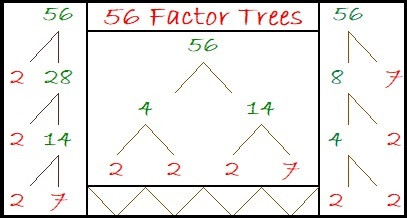# 55 and Square Roots for Third Grade

55 is a composite number. 55 = 1 x 55 or 5 x 11. Factors of 55: 1, 5, 11, 55. Prime factorization: 55 = 5 x 11.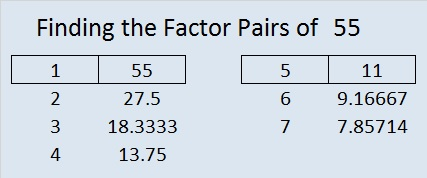Sometimes 55 is a clue in the FIND THE FACTORS 1 – 12 puzzles. Even though it has other factors, the puzzle only uses 5 x 11 = 55.

——————————————————————————————-

What is the square root of a number? If you had a square made with 9 squares, how many squares would be on each side?Even a third grader can count 3 squares on each side. That is one way to explain why √9 = 3 to someone so young. Here’s another explanation:

1. 1 x 1 = 1, so √1 = 1
2. 2 x 2 = 4, so √4 = 2
3. 3 x 3 = 9, so √9 = 3
4. 4 x 4 = 16, so √16 = 4
5. 5 x 5 = 25, so √25 = 5
6. 6 x 6 = 36, so √36 = 6
7. 7 x 7 = 49, so √49 = 7
8. 8 x 8 = 64, so √64 = 8
9. 9 x 9 = 81, so √81 = 9
10. 10 x 10 = 100, so √100 = 10

These are the kinds of square roots that third grade students may need to know. When they get older they will learn about other square roots like √2 ≈ ±1.414, but third graders don’t need to worry about those yet.

On January 24 someone hurriedly typed “aquare roots for third grade” into a search engine, and a post from this blog came up. The person took ONE quick look and closed the post and the blog just as hurriedly. I’m not exactly sure what aquare or square roots for third grade means, but I felt bad that the blog disappointed this unknown person. I thought, “That person MAY have been searching for something that actually might be on the blog SOMEWHERE…..Perhaps a puzzle with perfect square clues would have been a welcome sight.” Hey, I can dream, can’t I?

To solve today’s puzzle (It actually has perfect square clues!), you will have to take the square roots of the clues, and place those square roots in the top row as well as the first column. After you write down all those square roots, figure out what numbers go in the empty cells so that the entire puzzle works as a multiplication table.These square roots may or may not be the square roots for third grade that person was looking for. Nevertheless, I have personally seen several bright THIRD grade students solve similar perfect square puzzles that I’ve made. This week’s puzzles are available in an excel file here. If you have a spreadsheet program on your computer, you can access it. If you enable editing in excel, you can type your answers directly onto the puzzle, and you can also easily print the puzzles. Here are the factors for last week’s level 1 puzzle:# 54 and How Many Squares Are in This Puzzle?

How many squares are in this puzzle? Finding that answer is too tedious for me to pursue. There is 1 square that is bigger than all the rest, 169 of the smallest size squares, and some different number between 1 and 169 for each size square in between. Solving the actual puzzle will actually be less work and more fun. The actual puzzle challenges you to write the numbers 1 through 12 in the top row and again in the first column so that the answers you write and the clues inside the puzzle work together as a multiplication table. Use logic to find the unique solution to the puzzle.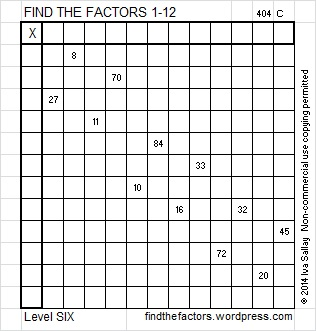This week’s puzzles are available in an excel file here. If you have a spreadsheet program on your computer, you can access it. If you enable editing in excel, you can type your answers directly onto the puzzle, and you can also easily print the puzzles. Here are the factors from last week’s level 6 hook-shaped puzzle:Here is one way those factors can be found using logic.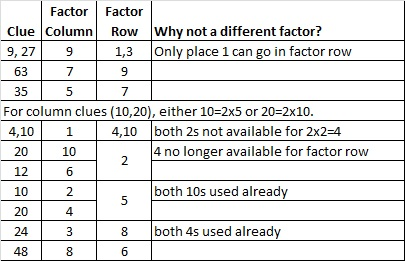54 is a composite number. 54 = 1 x 54, 2 x 27, 3 x 18, or 6 x 9. Factors of 54: 1, 2, 3, 6, 9, 18, 27, 54. Prime factorization: 54 = 2 x 3 x 3 x 3, which can also be written 2 x 3³.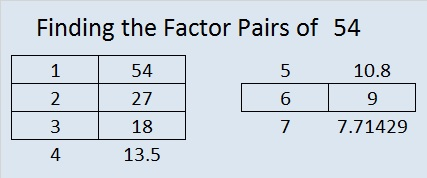Sometimes 54 is a clue in the FIND THE FACTORS puzzles. Even though it has other factors, the only multiplication fact the puzzle uses is 6 x 9 = 54.

# 50 and Multiples

Multiples and factors are related.

For example, 50 is a multiple of all these numbers: 1, 2, 5, 10, 25, and 50.

And 1, 2, 5, 10, 25, and 50 are all factors of 50.

50 is a composite number. 50 = 1 x 50, 2 x 25, or 5 x 10. Factors of 50: 1, 2, 5, 10, 25, 50. Prime factorization: 50 = 2 x 5 x 5, which can also be written 2 x 5².

The first few multiples of 50 are 50, 100, 150, 200, 250, 300, and so on…

If the difference between factors and multiples is confusing, this poster should help. Thanks to Resourceaholic for mentioning this great resource in one of her posts.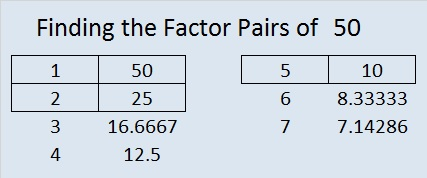Sometimes 50 is a clue in the FIND THE FACTORS puzzles. Even though it has other factors, we only use 50 = 5 x 10 to fill in the table.

Each of the clues inside this puzzle are MULTIPLES of a number from 1 to 12. Can you write every number from 1 to 12 in the top row as well as in the first column so that the clues are multiples of the numbers that you write?This week’s puzzles are available in an excel file here. If you have a spreadsheet program on your computer, you can access it. If you enable editing in excel, you can type your answers directly onto the puzzle, and you can also easily print the puzzles.

Here are the factors to last week’s level 4 puzzle:The chart below shows one possible way to arrive logically at the solution.